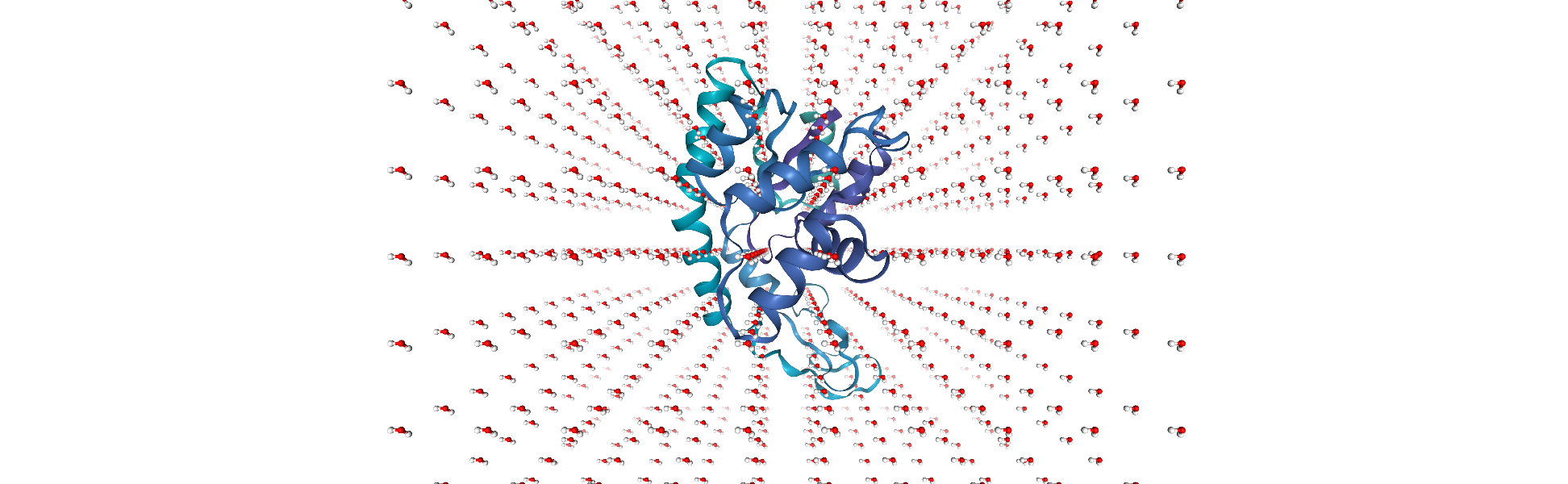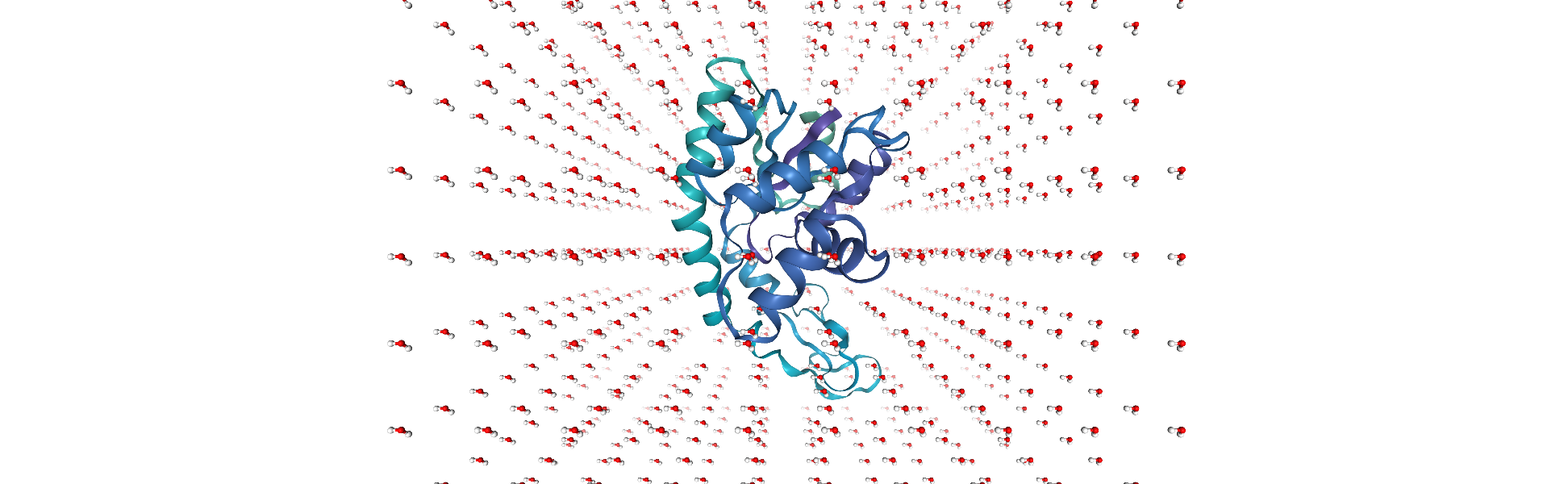# Constructing, modifying, and adding to a Universe

MDAnalysis version: ≥ 0.20.1

Last updated: December 2022 with MDAnalysis 2.4.0-dev0

Sometimes you may want to construct a Universe from scratch, or add attributes that are not read from a file. For example, you may want to group a Universe into chains, or create custom segments for protein domains.

In this tutorial we:

• create a Universe consisting of water molecules

• merge this with a protein Universe loaded from a file

• create custom segments labeling protein domains

Throughout this tutorial we will include cells for visualising Universes with the NGLView library. However, these will be commented out, and we will show the expected images generated instead of the interactive widgets.

:

import MDAnalysis as mda
from MDAnalysis.tests.datafiles import PDB_small
import numpy as np
from IPython.core.display import Image

import warnings
# suppress some MDAnalysis warnings when writing PDB files
warnings.filterwarnings('ignore')

print("Using MDAnalysis version", mda.__version__)

# Optionally, use NGLView to interactively view your trajectory
import nglview as nv
print("Using NGLView version", nv.__version__)

Using MDAnalysis version 2.6.0-dev0

Using NGLView version 3.0.3


## Creating and populating a Universe with water

### Creating a blank Universe

The Universe.empty() method creates a blank Universe. The natoms (int) argument must be included. Optional arguments are:

• n_residues (int): number of residues

• n_segments (int): number of segments

• atom_resindex (list): list of resindices for each atom

• residue_segindex (list): list of segindices for each residue

• trajectory (bool): whether to attach a MemoryReader trajectory (default False)

• velocities (bool): whether to include velocities in the trajectory (default False)

• forces (bool): whether to include forces in the trajectory (default False)

We will create a Universe with 1000 water molecules.

:

n_residues = 1000
n_atoms = n_residues * 3

# create resindex list
resindices = np.repeat(range(n_residues), 3)
assert len(resindices) == n_atoms
print("resindices:", resindices[:10])

# all water molecules belong to 1 segment
segindices =  * n_residues
print("segindices:", segindices[:10])

resindices: [0 0 0 1 1 1 2 2 2 3]
segindices: [0, 0, 0, 0, 0, 0, 0, 0, 0, 0]

:

# create the Universe
sol = mda.Universe.empty(n_atoms,
n_residues=n_residues,
atom_resindex=resindices,
residue_segindex=segindices,
trajectory=True) # necessary for adding coordinates
sol

:

<Universe with 3000 atoms>


There isn’t much we can do with our current Universe because MDAnalysis has no information on the particle elements, positions, etc. We can add relevant information manually using TopologyAttrs.

names

:

sol.add_TopologyAttr('name', ['O', 'H1', 'H2']*n_residues)
sol.atoms.names

:

array(['O', 'H1', 'H2', ..., 'O', 'H1', 'H2'], dtype=object)


elements (“types”)

Elements are typically contained in the type topology attribute.

:

sol.add_TopologyAttr('type', ['O', 'H', 'H']*n_residues)
sol.atoms.types

:

array(['O', 'H', 'H', ..., 'O', 'H', 'H'], dtype=object)


residue names (“resnames”)

:

sol.add_TopologyAttr('resname', ['SOL']*n_residues)
sol.atoms.resnames

:

array(['SOL', 'SOL', 'SOL', ..., 'SOL', 'SOL', 'SOL'], dtype=object)


residue counter (“resids”)

:

sol.add_TopologyAttr('resid', list(range(1, n_residues+1)))
sol.atoms.resids

:

array([   1,    1,    1, ..., 1000, 1000, 1000])


segment/chain names (“segids”)

:

sol.add_TopologyAttr('segid', ['SOL'])
sol.atoms.segids

:

array(['SOL', 'SOL', 'SOL', ..., 'SOL', 'SOL', 'SOL'], dtype=object)


Positions can simply be assigned, without adding a topology attribute.

The O-H bond length in water is around 0.96 Angstrom, and the bond angle is 104.45°. We can first obtain a set of coordinates for one molecule, and then translate it for every water molecule.

:

# coordinates obtained by building a molecule in the program IQMol
h2o = np.array([[ 0,        0,       0      ],  # oxygen
[ 0.95908, -0.02691, 0.03231],  # hydrogen
[-0.28004, -0.58767, 0.70556]]) # hydrogen

:

grid_size = 10
spacing = 8

coordinates = []

# translating h2o coordinates around a grid
for i in range(n_residues):
x = spacing * (i % grid_size)
y = spacing * ((i // grid_size) % grid_size)
z = spacing * (i // (grid_size * grid_size))

xyz = np.array([x, y, z])

coordinates.extend(h2o + xyz.T)

print(coordinates[:10])

[array([0., 0., 0.]), array([ 0.95908, -0.02691,  0.03231]), array([-0.28004, -0.58767,  0.70556]), array([8., 0., 0.]), array([ 8.95908, -0.02691,  0.03231]), array([ 7.71996, -0.58767,  0.70556]), array([16.,  0.,  0.]), array([16.95908, -0.02691,  0.03231]), array([15.71996, -0.58767,  0.70556]), array([24.,  0.,  0.])]

:

coord_array = np.array(coordinates)
assert coord_array.shape == (n_atoms, 3)
sol.atoms.positions = coord_array


We can view the atoms with NGLView, a library for visualising molecules. It guesses bonds based on distance.

:

# sol_view = nv.show_mdanalysis(sol)
# sol_view.center()
# sol_viewCurrently, the sol universe doesn’t contain any bonds.

:

assert not hasattr(sol, 'bonds')


They can be important for defining ‘fragments’, which are groups of atoms where every atom is connected by a bond to another atom in the group (i.e. what is commonly called a molecule). You can pass a list of tuples of atom indices to add bonds as a topology attribute.

:

bonds = []
for o in range(0, n_atoms, 3):
bonds.extend([(o, o+1), (o, o+2)])

bonds[:10]

:

[(0, 1),
(0, 2),
(3, 4),
(3, 5),
(6, 7),
(6, 8),
(9, 10),
(9, 11),
(12, 13),
(12, 14)]

:

sol.add_TopologyAttr('bonds', bonds)
sol.bonds

:

<TopologyGroup containing 2000 bonds>


The bonds associated with each atom or the bonds within an AtomGroup can be accessed with the bonds attribute:

:

print(sol.atoms.bonds)
print(sol.atoms[-10:].bonds)

<TopologyGroup containing 2 bonds>
<TopologyGroup containing 7 bonds>


## Merging with a protein

Now we can merge the water with a protein to create a combined system by using MDAnalysis.Merge to combine AtomGroup instances.

:

protein = mda.Universe(PDB_small)

:

# protein_view = nv.show_mdanalysis(protein)
# protein_viewWe will translate the centers of both systems to the origin, so they can overlap in space.

:

cog = sol.atoms.center_of_geometry()
print('Original solvent center of geometry: ', cog)
sol.atoms.positions -= cog
cog2 = sol.atoms.center_of_geometry()
print('New solvent center of geometry: ', cog2)

Original solvent center of geometry:  [36.22634681 35.79514029 36.24595657]
New solvent center of geometry:  [ 2.78155009e-07 -1.27156576e-07  3.97364299e-08]

:

cog = protein.atoms.center_of_geometry()
print('Original solvent center of geometry: ', cog)
protein.atoms.positions -= cog
cog2 = protein.atoms.center_of_geometry()
print('New solvent center of geometry: ', cog2)

Original solvent center of geometry:  [-3.66508082  9.60502842 14.33355791]
New solvent center of geometry:  [8.30580288e-08 3.49225059e-08 2.51332265e-08]

:

combined = mda.Merge(protein.atoms, sol.atoms)

:

# combined_view = nv.show_mdanalysis(combined)
# combined_viewUnfortunately, some water molecules overlap with the protein. We can create a new AtomGroup containing only the molecules where every atom is further away than 6 angstroms from the protein.

:

no_overlap = combined.select_atoms("same resid as (not around 6 protein)")


With this AtomGroup, we can then construct a new Universe.

:

u = mda.Merge(no_overlap)

:

# no_overlap_view = nv.show_mdanalysis(u)
# no_overlap_viewOften you may want to assign atoms to a segment or chain – for example, adding segment IDs to a PDB file. This requires adding a new Segment with Universe.add_Segment.

Adenylate kinase has three domains: CORE, NMP, and LID. As shown in the picture below, these have the residues:

• CORE: residues 1-29, 60-121, 160-214 (gray)

• NMP: residues 30-59 (blue)

• LID: residues 122-159 (yellow):

u.segments.segids

:

array(['4AKE', 'SOL'], dtype=object)


On examining the Universe, we can see that the protein and solvent are already divided into two segments: protein (‘4AKE’) and solvent (‘SOL’). We will add three more segments (CORE, NMP, and LID) and assign atoms to them.

First, add a Segment to the Universe with a segid. It will be empty:

:

core_segment = u.add_Segment(segid='CORE')
core_segment.atoms

:

<AtomGroup with 0 atoms>


Residues can’t be broken across segments. To put atoms in a segment, assign the segments attribute of their residues:

:

core_atoms = u.select_atoms('resid 1:29 or resid 60:121 or resid 160-214')
core_atoms.residues.segments = core_segment
core_segment.atoms

:

<AtomGroup with 2744 atoms>

:

nmp_segment = u.add_Segment(segid='NMP')

nmp_atoms = u.select_atoms('resid 30:59')
nmp_atoms.residues.segments = nmp_segment

lid_atoms = u.select_atoms('resid 122:159')
lid_atoms.residues.segments = lid_segment


As of MDAnalysis 2.1.0, PDBs use the chainID TopologyAttr for the chainID column. If it is missing, it uses a placeholder “X” value instead of the segid. We therefore must manually set that ourselves to visualise the protein in NGLView.

:

# add the topologyattr to the universe
core_segment.atoms.chainIDs = "C"
nmp_segment.atoms.chainIDs = "N"
lid_segment.atoms.chainIDs = "L"


We can check that we have the correct domains by visualising the protein.

:

# domain_view = nv.show_mdanalysis(u)
# domain_view## Tiling into a larger Universe

We can use MDAnalysis to tile out a smaller Universe into a bigger one, similarly to editconf in GROMACS. To start off, we need to figure out the box size. The default in MDAnalysis is a zero vector. The first three numbers represent the length of each axis, and the last three represent the alpha, beta, and gamma angles of a triclinic box.

:

print(u.dimensions)

None


We know that our system is cubic in shape, so we can assume angles of 90°. The difference between the lowest and highest x-axis positions is roughly 73 Angstroms.

:

max(u.atoms.positions[:, 0]) - min(u.atoms.positions[:, 0])

:

73.23912


So we can set our dimensions.

:

u.dimensions = [73, 73, 73, 90, 90, 90]


To tile out a Universe, we need to copy it and translate the atoms by the box dimensions. We can then merge the cells into one large Universe and assign new dimensions.

:

def tile_universe(universe, n_x, n_y, n_z):
box = universe.dimensions[:3]
copied = []
for x in range(n_x):
for y in range(n_y):
for z in range(n_z):
u_ = universe.copy()
move_by = box*(x, y, z)
u_.atoms.translate(move_by)
copied.append(u_.atoms)

new_universe = mda.Merge(*copied)
new_box = box*(n_x, n_y, n_z)
new_universe.dimensions = list(new_box) + *3
return new_universe


Here is a 2 x 2 x 2 version of our original unit cell:

:

tiled = tile_universe(u, 2, 2, 2)

:

# tiled_view = nv.show_mdanalysis(tiled)
# tiled_view## Acknowledgments

The Universe tiling code was modified from @richardjgowers’s gist on the issue in 2016.